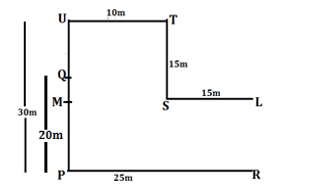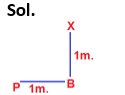# Direction Sense Based for IBPS, SBI, RBI, RRBs: Quiz 5

## Direction Sense Based for IBPS, SBI, RBI, RRBs: Quiz 5

Direction Sense Based plays a significant role in Reasoning Ability Section of banking exams such as IBPS, SBI and RBI PO and Clerk. You will get at least 3 to 5 questions from Direction Based in one of IBPS, SBI and RBI PO & clerk prelims and Mains exam. So, aspirants should focus on Direction Sense Based questions in detail. Here, we are providing you with the Direction Sense Based questions quiz with the detailed solution so that you can easily prepare for Direction Based questions. We are providing here all-important latest pattern-based questions and Previous Year Questions of Direction Based of various Government Exam like IBPS, SBI, and RBI PO and Clerk exam. This Direction Sense Based quiz we are providing is free. Attempt this Direction Based quiz to practice important questions with answers and solutions. And score better in IBPS, SBI and RBI PO and Clerk exam.

Direction Sense Based Quiz to improve your Reasoning Ability for SBI Po & SBI clerk exam IBPS PO Reasoning, IBPS Clerk Reasoning, IBPS RRB Reasoning, LIC AAO, LIC Assistant and another competitive exam.

Direction (1-3): Read the information carefully and answer the question:

Point U is 10m north of point Q. Point T is 10m east of point U. Point S is 15m south of point T. Point P is 20m south of point Q. Point R is 25m east of point P. Point L is 15m east of point S. Point M is the midpoint of point U and P.

1. What is the distance between point L and R?

(a) 10m

(b) 15m

(c) 5m

(d) 20m

(e) 25m

Ans. b

Sol.1. In which direction is point T with respect to P?

(a) north-west

(b) south-west

(c) south-east

(d) north-east

(e) none of these

Ans. d

Sol.1. Which of the following points are inline?

(a) P, R, S

(b) Q, M, L

(c) U, S, T

(d) M, S, L

(e) Q, S, L

Ans. d

Sol.Directions (4-7): Read the following information carefully and answer the questions which follows.

Three persons P, Q, and R are standing in a park. They are standing in a straight line which is aligned in the North-South direction. They are standing at three different points A, B and C. Point B is equidistant from point A and C. They are facing either east or west direction. P started moving ahead from point A and reached point D after walking 3 meters. After that from point D, he turned right and moved two meters to reach point E where he stopped. Q started from moving ahead from point B and reached point F after walking three meters. Then from F, he turned towards right and moved four meters to reach point G from where he turned right and moved eight meters to reach point H. R started moving ahead from point C and after walking five meters, he reached point I. Then from point I he took a left turn and kept on walking ahead till he reached point H. Point F is to the west of point E. A is in north of point C.

1. In which direction is point G with respect to point I?

(a) North

(b) North-East

(c) North-West

(d) Cannot be determined

(e) None of these

Ans. c

Sol.1. What is the shortest distance between point G and B?

(a) 5 meters

(b) 4 meters

(c) 8 meters

(d) 9 meters

(e) None of these

Ans. a

Sol.1. What is the total distance travelled by R to reach point H?

(a) 8 meters

(b) 9 meters

(c) 11 meters

(d) 10 meters

(e) None of these

Ans. c

Sol.1. In which direction is point C with respect to point H?

(a) South

(b) South-East

(c) South-West

(d) Cannot be determined

(e) None of these

Ans. c

Sol.Directions (8-10): Each of the following questions is based on the following information:

A # B means B is at 1 metre to the right of A.

A \$ B means B is at 1 metre to the North of A.

A * B means B is at 1 metre to the left of A.

A @ B means B is at 1 metre to the south of A.

1. According to X @ B * P, P is in which direction with respect to X?

(a) North

(b) South

(c) North-East

(d) South-West

(e) None of these.

Ans. d1. According to M # N \$ T, T is in which direction with respect to M?

(a) North-West

(b) North-East

(c) South-West

(d) South-East

(e) None of these

Ans. b1. According to P # R \$ A * U, in which direction is U with respect to P?

(a) East

(b) West

(c) North

(d) South

(e) None of these.

Ans. c#### Aatma Nirbhar Reasoning Topic Wise Online Test Series

Recommended PDF’s for :

#### Most important PDF’s for Bank, SSC, Railway and Other Government Exam : Download PDF Now

AATMA-NIRBHAR Series- Static GK/Awareness Practice Ebook PDF Get PDF here
The Banking Awareness 500 MCQs E-book| Bilingual (Hindi + English) Get PDF here
AATMA-NIRBHAR Series- Banking Awareness Practice Ebook PDF Get PDF here
Computer Awareness Capsule 2.O Get PDF here
AATMA-NIRBHAR Series Quantitative Aptitude Topic-Wise PDF Get PDF here
AATMA-NIRBHAR Series Reasoning Topic-Wise PDF Get PDF Here
Memory Based Puzzle E-book | 2016-19 Exams Covered Get PDF here
Caselet Data Interpretation 200 Questions Get PDF here
Puzzle & Seating Arrangement E-Book for BANK PO MAINS (Vol-1) Get PDF here
ARITHMETIC DATA INTERPRETATION 2.O E-book Get PDF here
3